The Simplified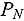(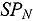) approximation is often used to model radiation transport phenomena, but it converges to the true solution of the transport equation only in one-dimensional slab geometry. In all other geometries, it incurs a model error that needs to be quantified. In this paper, we estimate the radiation transport model error due to the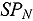approximation and employ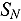transport solutions (with high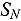order) as reference transport solutions. Because the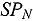solution does not contain the full angular information of the transport solution, an angular intensity must be reconstructed from the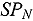solution in order to compute the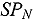model error. We propose two such reconstruction schemes. Model error estimates are given for various quantities of interests, i.e., scalar radiation intensity, radiation flux, and boundary leakage. An adjoint-based approach is proposed to evaluate the model error and is compared against forward and residual techniques. Two-dimensional numerical experiments are presented.